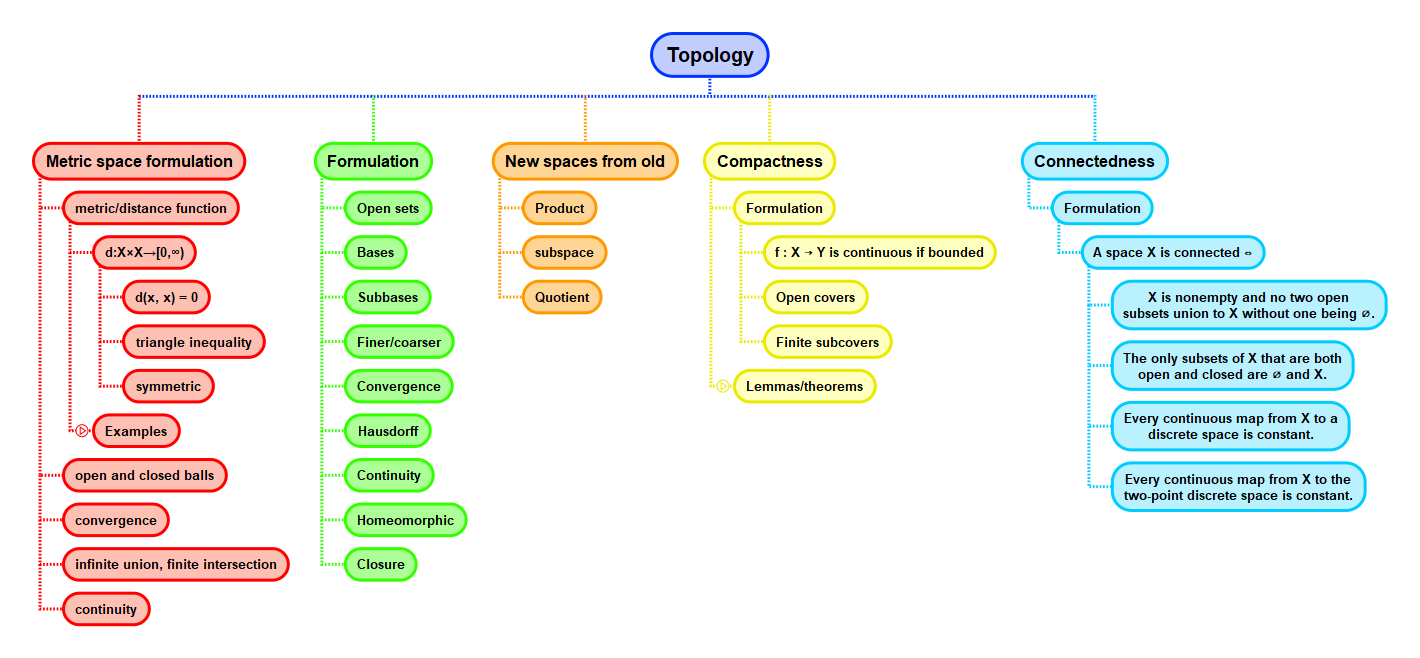Show Question
Math and science::Topology

# Connectedness. Definition

### Connectedness

Let $$X$$ be a topological space. A separation of $$X$$ is a pair $$U, V$$ of disjoint nonempty open subsets of $$X$$ whose union is $$X$$. The space $$X$$ is said to be connected iff there does not exist a separation of $$X$$.

This definition is from Munkres. Leinster's definition feels a little less intuitively concrete.

#### Connectedness, formulation 2 (or now a lemma)

A space $$X$$ is connected iff the only subsets of $$X$$ that are both open and closed in $$X$$ are the empty set and $$X$$ itself.

Again, from Munkres.

### Connectedness, motivation

Focus on two sets that union to create the set in question: if two sets union to the whole but are not "touching", then the set in question is disconnected. Intuitively, a space is said to be 'connected' if it is all in one piece—it does not fall naturally into two or more pieces. 'Naturally' here carries a major component of the sense of 'connected', as any topological space can be torn into multiple pieces in some way.

### Disconnected

The idea of a set being disconnected is possibly more intuitive than the idea of a set being connected. In $$\mathbb{R}$$, a set $$E \subseteq \mathbb{R}$$ is disconnected if it can be expressed as the union of two separated sets. Separated here means that the sets share no limit points ($$A$$ and $$B$$ are separated if $$\overline{A} \cap B$$ and $$A \cap \overline{B}$$ are empty). In other words, one can't find a sequence in $$A$$ whose limit is in $$B$$ and vise versa.

Yet another formulation is to say that $$E \subseteq \mathbb{R}$$ is disconnected iff for some $$a, b \in E$$ there is a $$c \in \mathbb{R}$$ but not in $$E$$ such that $$a < c < b$$. Push a negation into this statement to get back to the connected version: $$E$$ is connected iff for all $$a, b \in E$$, whenever $$a < c < b$$ for some $$c \in \mathbb{R}$$, then $$c \in E$$.

#### Tearing

Intuitively, why can't the real line $$\mathbb{R}$$ be separated naturally into two pieces? Consider the decomposition $$(-\infty, 0) \cup [0, \infty)$$: in what way is this separation not natural? While it might not appeal to everyone's intuition, this separation is considered forced because a limit point of $$(-\infty, 0)$$, zero, is not an element of $$(-\infty, 0)$$ but an element of the other piece $$[0, \infty)$$. In other words, the closure of one piece spills into or is contained or is intertwined in the other piece. Thus, naturally separating a space can be viewed from the lens of open and closed sets.

#### Natural separation, requirements

For a topological space $$X$$, a separation of $$X$$ into disjoint subsets is considered a natural separation into independent pieces $$U$$ and $$V$$ if no point in $$U$$ is a limit point of $$V$$, and vice versa. Equivalently, this means that $$Cl(U)$$ is disjoin from $$V$$, and vice versa, or equivalently, that both $$U$$ and $$V$$ are closed, or equivalently yet again, that both $$U$$ and $$V$$ are open.

Thus, we arrive at our definition of connectedness, by defining a space $$X$$ connected if the only natural separation of $$X$$ as described above is the trivial one, $$\emptyset$$ and $$X$$ itself.

#### Sufficiency of open sets

If disjoint open sets are sufficient to cover a space, then the space is not connected. Open sets don't contain their boundary limits, and two disjoint open sets must therefore not be touching. There is at least a point that exists in the sense of limits that is in neither of the open sets. In this sense, the space is disconnected.

## ContextMunkres, p146
Leinster, p68